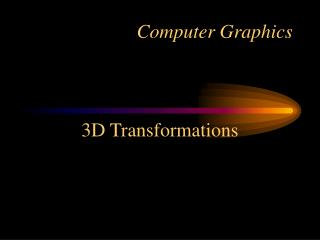Download PresentationComputer GraphicsComputer Graphics - PowerPoint PPT Presentation

Download PresentationComputer Graphics
An Image/Link below is provided (as is) to download presentation

Download Policy: Content on the Website is provided to you AS IS for your information and personal use and may not be sold / licensed / shared on other websites without getting consent from its author. While downloading, if for some reason you are not able to download a presentation, the publisher may have deleted the file from their server.

- - - - - - - - - - - - - - - - - - - - - - - - - - - E N D - - - - - - - - - - - - - - - - - - - - - - - - - - -
Presentation Transcript

1. Computer Graphics 3D Transformations

2. 3D Translation • Remembering 2D transformations -> 3x3 matrices, take a wild guess what happens to 3D transformations. T=(tx, ty, tz)

3. 3D Scale S=(sx, sy, sz)

4. 3D Rotations What does a rotation in 3D mean? Q: How do we specify a rotation? R=(rx, ry, rz, ) A: We give a vector to rotate about, and a theta that describes how much we rotate.  Q: Since 2D is sort of like a special case of 3D, what is the vector we’ve been rotating about in 2D?

5. Rotations about the Z axis What do you think the rotation matrix is for rotations about the z axis? R=(0,0,1,) 

6. Rotations about the X axis Let’s look at the other axis rotations R=(1,0,0,) 

7. Rotations about the Y axis R=(0,1,0,) 

8. Rotations around a principle axis

9.   Rotations for an arbitrary axis u Steps: 1. Normalize vector u 2. Compute  3. Compute  4. Create rotation matrix

10. Vector Normalization • Given a vector v, we want to create a unit vector that has a magnitude of 1 and has the same direction as v. Let’s do an example.

11. Computing the Rotation Matrix • 1. Normalize u ( the vector we are rotating around ) • 2. Compute Rx(x) • 3. Compute Ry(y) • 4. Generate Rotation Matrix

12. Rotations for an arbitrary axis • Unit vectors are made of direction cosines:

13. Rotations for an arbitrary axis

14. Rotations for an arbitrary axis

15. Rotation Matrix

16. Axis-Angle Rotations in OpenGL glRotatef( angle, x, y, z);

17. Euler Angles More intuitive: represent rotations by 3 angles, one for each axis glRotatef(anglex,1,0,0); glRotatef(angley,0,1,0); glRotatef(anglez,0,0,1); Think: if we have a torus unstranformed at the origin, what will the torus look like if you have anglex=90, angley=90, and anglez=90 Transformed

18. Gimbal Lock Rotate around these Initial orientation (x=blue, y=green, z = red) How can we gain altitude here?

19. Gimbal Lock in OpenGL What is the problem here? glRotatef(anglex,1,0,0); glRotatef(angley,0,1,0); glRotatef(anglez,0,0,1);

20. Avoiding Gimbal Lock: Quaternions • Quaternions represent 3D rotations in 4D using imaginary numbers – a 4-tuple Q = (w,x,y,z) • Convert Angle-axis -> Quaternion • (,x,y,z) -> ( cos( /2), xsin(/2), ysin(/2), zsin(/2) ) • Why quaternions? • No gimbal lock • Smooth interpolation between rotations (for animation)

21. Quaternions • Quaternions have rules for multiplication, inversion, etc • See: http://www.j3d.org/matrix_faq/matrfaq_latest.html • Typical usage: • Convert from euler, matrix, or angle-axis • Do rotations • Convert to angle-axis or matrix

22. What is going on here? frame1 frame3 frame2

23. gluLookAt Orients and positions the “camera” gluLookat( eyex, eyey, eyez, centerx, centery, centerz, upx,upy, upz); eye – the position of the camera in world coordinates center – the camera is pointed at this point up – the direction defined to be up for the camera

24. gluLookAt – in matrix form

25. u  Cross Products Given two vectors, the cross product returns a vector that is perpendicular to the plane of the two vectors and with magnitude equal to the area of the parallelogram formed by the two vectors.

26. Different Phases:Model Definition

27. Different Phases:Transformations

28. Different Phases:Projection

29. Different Phases:Projection

30. Different Phases:Rasterization

31. Different Phases:Scan Conversion

32. What are the steps needed?

33. Let’s Examine the Camera • If I gave you a world, and said I want to “render” it from another viewpoint, what information do I have to give you? • Position • Which way we are looking • Which way is “up” • Aspect Ratio • Field of View • Near and Far

34. Camera View Right View Up View Normal View Direction

35. Camera View Up View Right What are the vectors?

36. Graphics Pipeline So Far Object Object Coordinates Transformation Object -> World World World Coordinates Projection Xform World -> Projection Normalize Xform & Clipping Projection -> Normalized Camera Projection Coordinates Viewport Normalized Coordinates Viewport Transform Normalized -> Device Screen Device Coordinates

37. Transformation World->Camera View Right View Up View Normal View Direction

38. Transformation World->Camera View Right = u View Up = V View Direction = -N